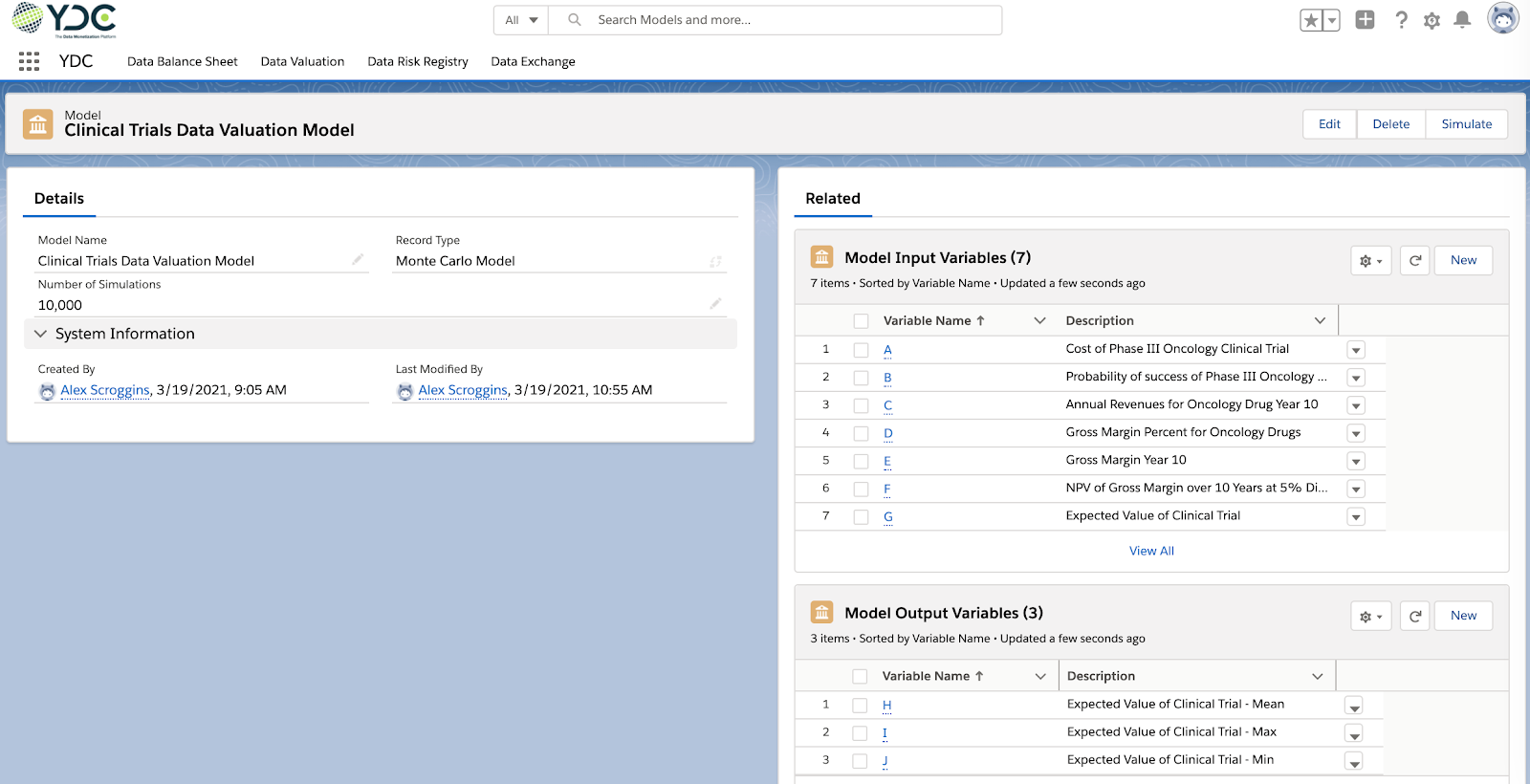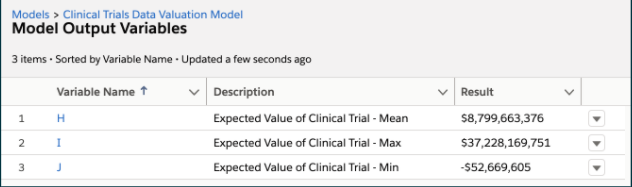Sunil Soares

# Blog: Valuation of Life Sciences Clinical Trial Data and Data Balance Sheet Using YDC

Clinical trials generate data that has an uncertain value. A trial may or may not be successful, and it may generate revenues that are uncertain. We leveraged YDC to develop a valuation model for Phase III clinical trials for an oncology (cancer) drug.

As a first step, we created a Clinical Trials Data Valuation Model in YDC (Figure 1). The model will be based on 10,000 Monte Carlo simulations.The model has the following input variables:

A. Cost of Phase III Oncology Clinical Trial
B. Probability of success of Phase III Oncology Clinical Trial
C. Annual Revenues for Oncology Drug Year 10
D. Gross Margin Percent for Oncology Drugs
E. Gross Margin Year 10 (C x D)
F. Net Present Value (NPV) of Gross Margin over 10 years at 5% Discount (based on E)
G. Expected Value of Clinical Trial [(B x F) – A]

The model has the following output variables:

H. Expected Value of Clinical Trial – Mean
I. Expected Value of Clinical Trial – Max
J. Expected Value of Clinical Trial – MinFigure 1: Clinical trials data valuation model in YDC
Each input variable in the model must have a Lower Bound, Upper Bound, and Most Likely value specified. We then ran 10,000 Monte Carlo simulations and produced output variables as summarized in Figure 2.Figure 2: Output variables associated with Monte Carlo simulation of clinical trials data valuation